# snssde1d()

Assume that we want to describe the following SDE:

Ito form3:

$\begin{equation}\label{eq:05} dX_{t} = \frac{1}{2}\theta^{2} X_{t} dt + \theta X_{t} dW_{t},\qquad X_{0}=x_{0} > 0 \end{equation}$

Stratonovich form: $\begin{equation}\label{eq:06} dX_{t} = \frac{1}{2}\theta^{2} X_{t} dt +\theta X_{t} \circ dW_{t},\qquad X_{0}=x_{0} > 0 \end{equation}$

In the above $$f(t,x)=\frac{1}{2}\theta^{2} x$$ and $$g(t,x)= \theta x$$ ($$\theta > 0$$), $$W_{t}$$ is a standard Wiener process. To simulate this models using snssde1d() function we need to specify:

• The drift and diffusion coefficients as R expressions that depend on the state variable x and time variable t.
• The number of simulation steps N=1000 (by default: N=1000).
• The number of the solution trajectories to be simulated by M=1000 (by default: M=1).
• The initial conditions t0=0, x0=10 and end time T=1 (by default: t0=0, x0=0 and T=1).
• The integration step size Dt=0.001 (by default: Dt=(T-t0)/N).
• The choice of process types by the argument type="ito" for Ito or type="str" for Stratonovich (by default type="ito").
• The numerical method to be used by method (by default method="euler").
R> set.seed(1234, kind = "L'Ecuyer-CMRG")
R> theta = 0.5
R> f <- expression( (0.5*theta^2*x) )
R> g <- expression( theta*x )
R> mod1 <- snssde1d(drift=f,diffusion=g,x0=10,M=1000,type="ito") # Using Ito
R> mod2 <- snssde1d(drift=f,diffusion=g,x0=10,M=1000,type="str") # Using Stratonovich
R> mod1
Itô Sde 1D:
| dX(t) = (0.5 * theta^2 * X(t)) * dt + theta * X(t) * dW(t)
Method:
| Euler scheme with order 0.5
Summary:
| Size of process   | N  = 1001.
| Number of simulation  | M  = 1000.
| Initial value     | x0 = 10.
| Time of process   | t in [0,1].
| Discretization    | Dt = 0.001.
R> mod2
Stratonovich Sde 1D:
| dX(t) = (0.5 * theta^2 * X(t)) * dt + theta * X(t) o dW(t)
Method:
| Euler scheme with order 0.5
Summary:
| Size of process   | N  = 1001.
| Number of simulation  | M  = 1000.
| Initial value     | x0 = 10.
| Time of process   | t in [0,1].
| Discretization    | Dt = 0.001.

Using Monte-Carlo simulations, the following statistical measures (S3 method) for class snssde1d() can be approximated for the $$X_{t}$$ process at any time $$t$$:

• The expected value $$\text{E}(X_{t})$$ at time $$t$$, using the command mean.
• The variance $$\text{Var}(X_{t})$$ at time $$t$$, using the command moment with order=2 and center=TRUE.
• The median $$\text{Med}(X_{t})$$ at time $$t$$, using the command Median.
• The mode $$\text{Mod}(X_{t})$$ at time $$t$$, using the command Mode.
• The quartile of $$X_{t}$$ at time $$t$$, using the command quantile.
• The maximum and minimum of $$X_{t}$$ at time $$t$$, using the command min and max.
• The skewness and the kurtosis of $$X_{t}$$ at time $$t$$, using the command skewness and kurtosis.
• The coefficient of variation (relative variability) of $$X_{t}$$ at time $$t$$, using the command cv.
• The central moments up to order $$p$$ of $$X_{t}$$ at time $$t$$, using the command moment.
• The empirical $$\alpha \%$$ confidence interval of expected value $$\text{E}(X_{t})$$ at time $$t$$ (from the $$2.5th$$ to the $$97.5th$$ percentile), using the command bconfint.
• The result summaries of the results of Monte-Carlo simulation at time $$t$$, using the command summary.

The summary of the results of mod1 and mod2 at time $$t=1$$ of class snssde1d() is given by:

R> summary(mod1, at = 1)

Monte-Carlo Statistics for X(t) at time t = 1

Mean                  11.21041
Variance              37.34360
Median                 9.95235
Mode                   8.03077
First quartile         7.00050
Third quartile        13.84224
Minimum                2.24849
Maximum               47.23754
Skewness               1.78370
Kurtosis               8.23099
Coef-variation         0.54511
3th-order moment     407.04925
4th-order moment   11478.47403
5th-order moment  299393.26186
6th-order moment 9011343.65855
R> summary(mod2, at = 1)

Monte-Carlo Statistics for X(t) at time t = 1

Mean                   12.8797
Variance               54.2186
Median                 11.3893
Mode                    9.8106
First quartile          8.0782
Third quartile         15.3722
Minimum                 2.0887
Maximum                72.4702
Skewness                2.2451
Kurtosis               11.6921
Coef-variation          0.5717
3th-order moment      896.3033
4th-order moment    34370.6613
5th-order moment  1387912.1394
6th-order moment 65677123.6792

Hence we can just make use of the rsde1d() function to build our random number generator for the conditional density of the $$X_{t}|X_{0}$$ ($$X_{t}^{\text{mod1}}| X_{0}$$ and $$X_{t}^{\text{mod2}}|X_{0}$$) at time $$t = 1$$.

R> x1 <- rsde1d(object = mod1, at = 1)  # X(t=1) | X(0)=x0 (Ito SDE)
R> x2 <- rsde1d(object = mod2, at = 1)  # X(t=1) | X(0)=x0 (Stratonovich SDE)
R> head(data.frame(x1,x2),n=5)
       x1      x2
1  8.7078  9.3026
2  7.8980  7.9105
3 10.7227 15.5981
4 10.4143 12.2105
5  4.5190  4.7178

The function dsde1d() can be used to show the Approximate transitional density for $$X_{t}|X_{0}$$ at time $$t-s=1$$ with log-normal curves:

R> mu1 = log(10); sigma1= sqrt(theta^2)  # log mean and log variance for mod1
R> mu2 = log(10)-0.5*theta^2 ; sigma2 = sqrt(theta^2) # log mean and log variance for mod2
R> AppdensI <- dsde1d(mod1, at = 1)
R> AppdensS <- dsde1d(mod2, at = 1)
R> plot(AppdensI , dens = function(x) dlnorm(x,meanlog=mu1,sdlog = sigma1))
R> plot(AppdensS , dens = function(x) dlnorm(x,meanlog=mu2,sdlog = sigma2))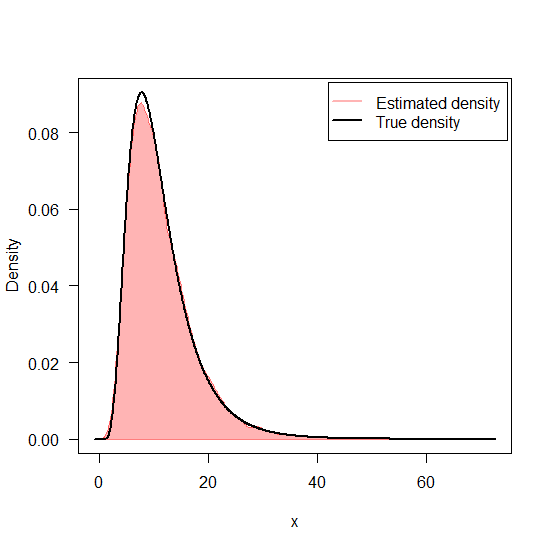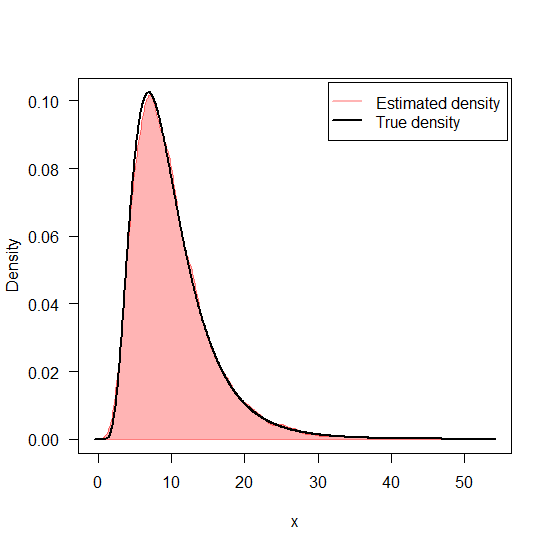In Figure 2, we present the flow of trajectories, the mean path (red lines) of solution of and , with their empirical $$95\%$$ confidence bands, that is to say from the $$2.5th$$ to the $$97.5th$$ percentile for each observation at time $$t$$ (blue lines):

R> ## Ito
R> plot(mod1,ylab=expression(X^mod1))
R> lines(time(mod1),apply(mod1$X,1,mean),col=2,lwd=2) R> lines(time(mod1),apply(mod1$X,1,bconfint,level=0.95)[1,],col=4,lwd=2)
R> lines(time(mod1),apply(mod1$X,1,bconfint,level=0.95)[2,],col=4,lwd=2) R> legend("topleft",c("mean path",paste("bound of", 95,"% confidence")),inset = .01,col=c(2,4),lwd=2,cex=0.8) R> ## Stratonovich R> plot(mod2,ylab=expression(X^mod2)) R> lines(time(mod2),apply(mod2$X,1,mean),col=2,lwd=2)
R> lines(time(mod2),apply(mod2$X,1,bconfint,level=0.95)[1,],col=4,lwd=2) R> lines(time(mod2),apply(mod2$X,1,bconfint,level=0.95)[2,],col=4,lwd=2)
R> legend("topleft",c("mean path",paste("bound of",95,"% confidence")),col=c(2,4),inset =.01,lwd=2,cex=0.8)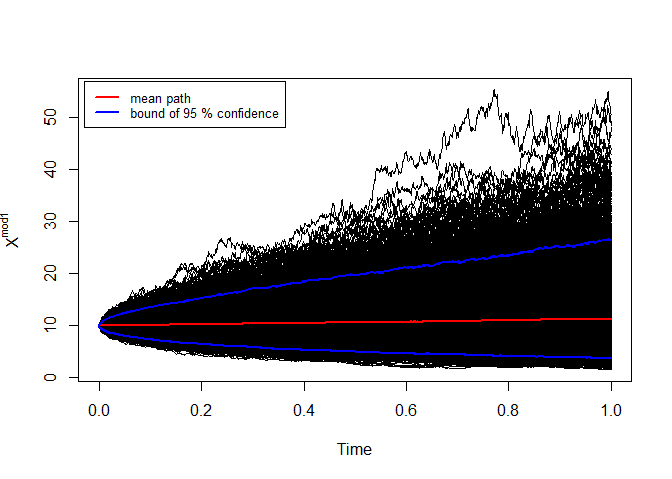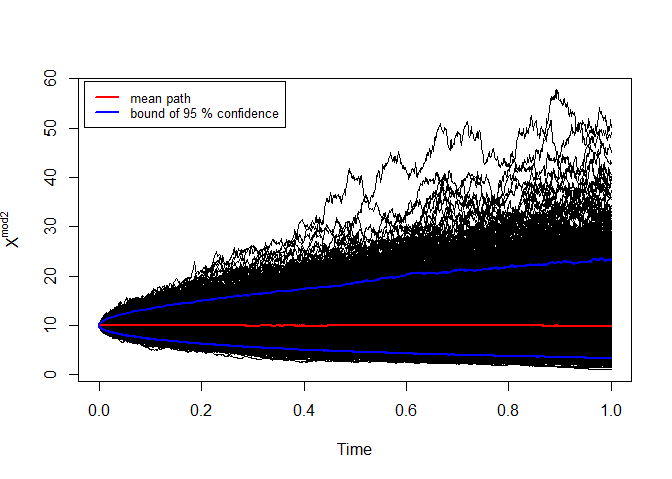# snssde2d()

The following $$2$$-dimensional SDE’s with a vector of drift and matrix of diffusion coefficients:

Ito form: $\begin{equation}\label{eq:09} \begin{cases} dX_t = f_{x}(t,X_{t},Y_{t}) dt + g_{x}(t,X_{t},Y_{t}) dW_{1,t}\\ dY_t = f_{y}(t,X_{t},Y_{t}) dt + g_{y}(t,X_{t},Y_{t}) dW_{2,t} \end{cases} \end{equation}$

Stratonovich form: $\begin{equation}\label{eq:10} \begin{cases} dX_t = f_{x}(t,X_{t},Y_{t}) dt + g_{x}(t,X_{t},Y_{t}) \circ dW_{1,t}\\ dY_t = f_{y}(t,X_{t},Y_{t}) dt + g_{y}(t,X_{t},Y_{t}) \circ dW_{2,t} \end{cases} \end{equation}$ where $$(W_{1,t}, W_{2,t})$$ are a two independent standard Wiener process if corr = NULL. To simulate $$2d$$ models using snssde2d() function we need to specify:

• The drift (2d) and diffusion (2d) coefficients as R expressions that depend on the state variable x, y and time variable t.
• corr the correlation structure of two standard Wiener process $$(W_{1,t},W_{2,t})$$; must be a real symmetric positive-definite square matrix of dimension $$2$$ (default: corr=NULL).
• The number of simulation steps N (default: N=1000).
• The number of the solution trajectories to be simulated by M (default: M=1).
• The initial conditions t0, x0 and end time T (default: t0=0, x0=c(0,0) and T=1).
• The integration step size Dt (default: Dt=(T-t0)/N).
• The choice of process types by the argument type="ito" for Ito or type="str" for Stratonovich (default type="ito").
• The numerical method to be used by method (default method="euler").

## Ornstein-Uhlenbeck process and its integral

The Ornstein-Uhlenbeck (OU) process has a long history in physics. Introduced in essence by Langevin in his famous 1908 paper on Brownian motion, the process received a more thorough mathematical examination several decades later by Uhlenbeck and Ornstein (1930). The OU process is understood here to be the univariate continuous Markov process $$X_t$$. In mathematical terms, the equation is written as an Ito equation: $\begin{equation}\label{eq016} dX_t = -\frac{1}{\mu} X_t dt + \sqrt{\sigma} dW_t,\quad X_{0}=x_{0} \end{equation}$ In these equations, $$\mu$$ and $$\sigma$$ are positive constants called, respectively, the relaxation time and the diffusion constant. The time integral of the OU process $$X_t$$ (or indeed of any process $$X_t$$) is defined to be the process $$Y_t$$ that satisfies: $\begin{equation}\label{eq017} Y_{t} = Y_{0}+\int X_{t} dt \Leftrightarrow dY_t = X_{t} dt ,\quad Y_{0}=y_{0} \end{equation}$ $$Y_t$$ is not itself a Markov process; however, $$X_t$$ and $$Y_t$$ together comprise a bivariate continuous Markov process. We wish to find the solutions $$X_t$$ and $$Y_t$$ to the coupled time-evolution equations: $\begin{equation}\label{eq018} \begin{cases} dX_t = -\frac{1}{\mu} X_t dt + \sqrt{\sigma} dW_t\\ dY_t = X_{t} dt \end{cases} \end{equation}$

We simulate a flow of $$1000$$ trajectories of $$(X_{t},Y_{t})$$, with integration step size $$\Delta t = 0.01$$, and using second Milstein method.

R> set.seed(1234, kind = "L'Ecuyer-CMRG")
R> x0=5;y0=0
R> mu=3;sigma=0.5
R> fx <- expression(-(x/mu),x)
R> gx <- expression(sqrt(sigma),0)
R> mod2d <- snssde2d(drift=fx,diffusion=gx,Dt=0.01,M=1000,x0=c(x0,y0),method="smilstein")
R> mod2d
Itô Sde 2D:
| dX(t) = -(X(t)/mu) * dt + sqrt(sigma) * dW1(t)
| dY(t) = X(t) * dt + 0 * dW2(t)
Method:
| Second-order Milstein scheme
Summary:
| Size of process   | N  = 1001.
| Number of simulation  | M  = 1000.
| Initial values    | (x0,y0) = (5,0).
| Time of process   | t in [0,10].
| Discretization    | Dt = 0.01.

The summary of the results of mod2d at time $$t=10$$ of class snssde2d() is given by:

R> summary(mod2d, at = 10)

For plotting in time (or in plane) using the command plot (plot2d), the results of the simulation are shown in Figure 3.

R> ## in time
R> plot(mod2d)
R> ## in plane (O,X,Y)
R> plot2d(mod2d,type="n")
R> points2d(mod2d,col=rgb(0,100,0,50,maxColorValue=255), pch=16)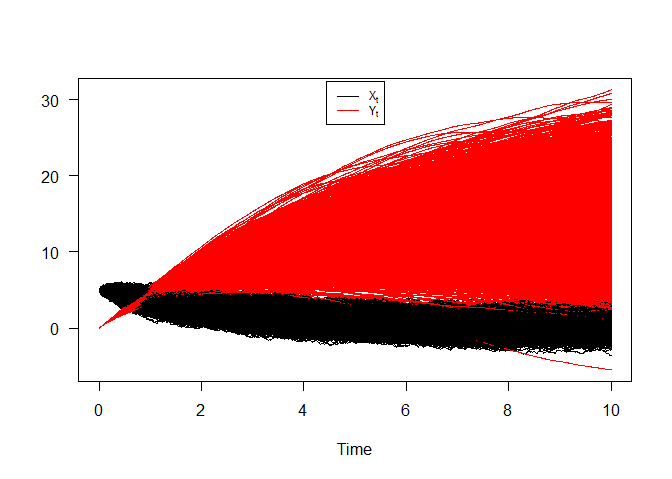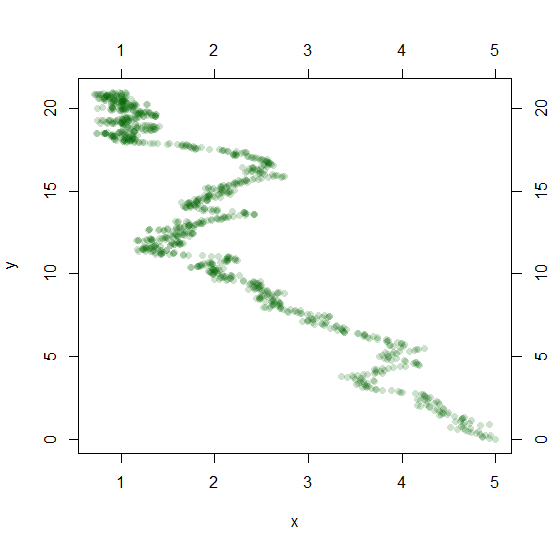Hence we can just make use of the rsde2d() function to build our random number for $$(X_{t},Y_{t})$$ at time $$t = 10$$.

R> out <- rsde2d(object = mod2d, at = 10)
R> head(out,n=3)
        x       y
1 0.32480 12.2224
2 0.91370  9.3092
3 0.49834 14.4406

The density of $$X_t$$ and $$Y_t$$ at time $$t=10$$ are reported using dsde2d() function, see e.g. Figure 4: the marginal density of $$X_t$$ and $$Y_t$$ at time $$t=10$$. For plotted in (x, y)-space with dim = 2. A contour and image plot of density obtained from a realization of system $$(X_{t},Y_{t})$$ at time t=10, see:

R> ## the marginal density
R> denM <- dsde2d(mod2d,pdf="M",at =10)
R> plot(denM, main="Marginal Density")
R> ## the Joint density
R> denJ <- dsde2d(mod2d, pdf="J", n=100,at =10)
R> plot(denJ,display="contour",main="Bivariate Transition Density at time t=10")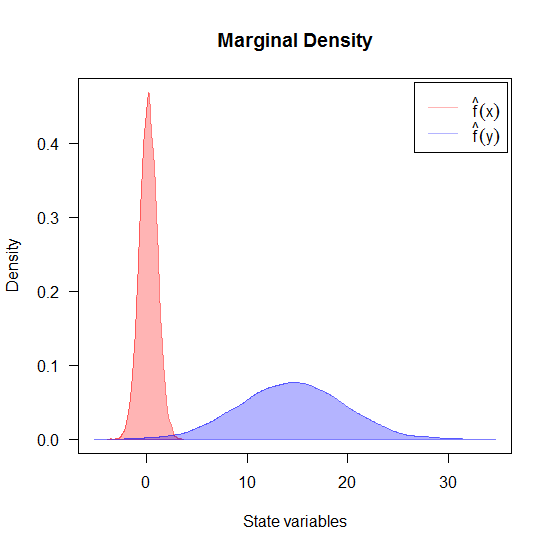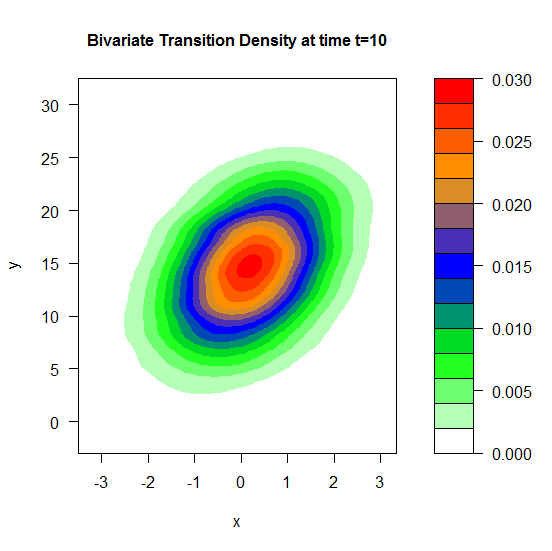A $$3$$D plot of the transition density at $$t=10$$ obtained with:

R> plot(denJ,display="persp",main="Bivariate Transition Density at time t=10")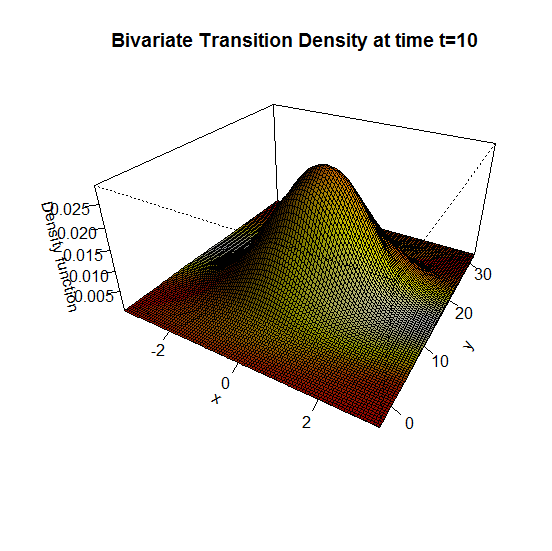Marginal and Joint density at time t=10

We approximate the bivariate transition density over the set transition horizons $$t\in [1,10]$$ by $$\Delta t = 0.005$$ using the code:

R> for (i in seq(1,10,by=0.005)){
+ plot(dsde2d(mod2d, at = i,n=100),display="contour",main=paste0('Transition Density \n t = ',i))
+ }

## The stochastic Van-der-Pol equation

The Van der Pol (1922) equation is an ordinary differential equation that can be derived from the Rayleigh differential equation by differentiating and setting $$\dot{x}=y$$, see Naess and Hegstad (1994); Leung (1995) and for more complex dynamics in Van-der-Pol equation see Jing et al. (2006). It is an equation describing self-sustaining oscillations in which energy is fed into small oscillations and removed from large oscillations. This equation arises in the study of circuits containing vacuum tubes and is given by: $\begin{equation}\label{eq:12} \ddot{X}-\mu (1-X^{2}) \dot{X} + X = 0 \end{equation}$ where $$x$$ is the position coordinate (which is a function of the time $$t$$), and $$\mu$$ is a scalar parameter indicating the nonlinearity and the strength of the damping, to simulate the deterministic equation see Grayling (2014) for more details. Consider stochastic perturbations of the Van-der-Pol equation, and random excitation force of such systems by White noise $$\xi_{t}$$, with delta-type correlation function $$\text{E}(\xi_{t}\xi_{t+h})=2\sigma \delta (h)$$ $\begin{equation}\label{eq:13} \ddot{X}-\mu (1-X^{2}) \dot{X} + X = \xi_{t}, \end{equation}$ where $$\mu > 0$$ . It’s solution cannot be obtained in terms of elementary functions, even in the phase plane. The White noise $$\xi_{t}$$ is formally derivative of the Wiener process $$W_{t}$$. The representation of a system of two first order equations follows the same idea as in the deterministic case by letting $$\dot{x}=y$$, from physical equation we get the above system: $\begin{equation}\label{eq:14} \begin{cases} \dot{X} = Y \\ \dot{Y} = \mu \left(1-X^{2}\right) Y - X + \xi_{t} \end{cases} \end{equation}$ The system can mathematically explain by a Stratonovitch equations: $\begin{equation}\label{eq:15} \begin{cases} dX_{t} = Y_{t} dt \\ dY_{t} = \left(\mu (1-X^{2}_{t}) Y_{t} - X_{t}\right) dt + 2 \sigma \circ dW_{2,t} \end{cases} \end{equation}$

Implemente in R as follows, with integration step size $$\Delta t = 0.01$$ and using stochastic Runge-Kutta methods 1-stage.

R> set.seed(1234, kind = "L'Ecuyer-CMRG")
R> mu = 4; sigma=0.1
R> fx <- expression( y ,  (mu*( 1-x^2 )* y - x))
R> gx <- expression( 0 ,2*sigma)
R> mod2d <- snssde2d(drift=fx,diffusion=gx,N=10000,Dt=0.01,type="str",method="rk1")

For plotting (back in time) using the command plot, and plot2d in plane the results of the simulation are shown in Figure 6.

R> plot(mod2d,ylim=c(-8,8))   ## back in time
R> plot2d(mod2d)              ## in plane (O,X,Y)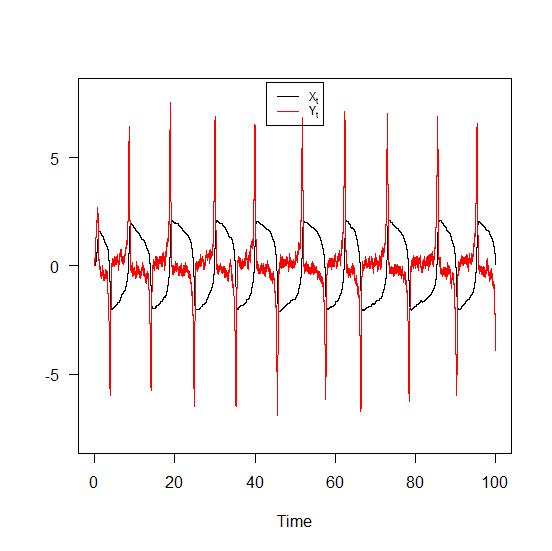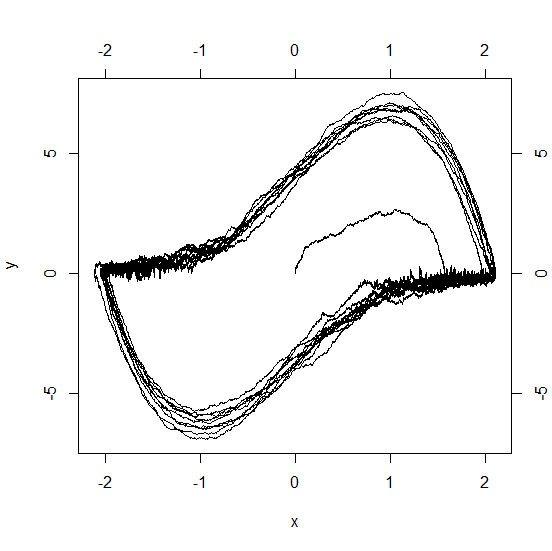## The Heston Model

Consider a system of stochastic differential equations:

$\begin{equation}\label{eq:115} \begin{cases} dX_{t} = \mu X_{t} dt + X_{t}\sqrt{Y_{t}} dB_{1,t}\\ dY_{t} = \nu (\theta-Y_{t}) dt + \sigma \sqrt{Y_{t}} dB_{2,t} \end{cases} \end{equation}$

Conditions to ensure positiveness of the volatility process are that $$2\nu \theta > \sigma^2$$, and the two Brownian motions $$(B_{1,t},B_{2,t})$$ are correlated. $$\Sigma$$ to describe the correlation structure, for example: $\Sigma= \begin{pmatrix} 1 & 0.3 \\ 0.3 & 2 \end{pmatrix}$

R> set.seed(1234, kind = "L'Ecuyer-CMRG")
R> mu = 1.2; sigma=0.1;nu=2;theta=0.5
R> fx <- expression( mu*x ,nu*(theta-y))
R> gx <- expression( x*sqrt(y) ,sigma*sqrt(y))
R> Sigma <- matrix(c(1,0.3,0.3,2),nrow=2,ncol=2) # correlation matrix
R> HM <- snssde2d(drift=fx,diffusion=gx,Dt=0.001,x0=c(100,1),corr=Sigma,M=1000)
R> HM
Itô Sde 2D:
| dX(t) = mu * X(t) * dt + X(t) * sqrt(Y(t)) * dB1(t)
| dY(t) = nu * (theta - Y(t)) * dt + sigma * sqrt(Y(t)) * dB2(t)
| Correlation structure:
1.0 0.3
0.3 2.0
Method:
| Euler scheme with order 0.5
Summary:
| Size of process   | N  = 1001.
| Number of simulation  | M  = 1000.
| Initial values    | (x0,y0) = (100,1).
| Time of process   | t in [0,1].
| Discretization    | Dt = 0.001.

Hence we can just make use of the rsde2d() function to build our random number for $$(X_{t},Y_{t})$$ at time $$t = 1$$.

R> out <- rsde2d(object = HM, at = 1)
R> head(out,n=3)
       x       y
1 181.42 0.56526
2 137.85 0.52612
3 243.57 0.62700

The density of $$X_t$$ and $$Y_t$$ at time $$t=1$$ are reported using dsde2d() function. See:

R> denJ <- dsde2d(HM,pdf="J",at =1,lims=c(-100,900,0.4,0.75))
R> plot(denJ,display="contour",main="Bivariate Transition Density at time t=10")
R> plot(denJ,display="persp",main="Bivariate Transition Density at time t=10")

# snssde3d()

The following $$3$$-dimensional SDE’s with a vector of drift and matrix of diffusion coefficients:

Ito form: $\begin{equation}\label{eq17} \begin{cases} dX_t = f_{x}(t,X_{t},Y_{t},Z_{t}) dt + g_{x}(t,X_{t},Y_{t},Z_{t}) dW_{1,t}\\ dY_t = f_{y}(t,X_{t},Y_{t},Z_{t}) dt + g_{y}(t,X_{t},Y_{t},Z_{t}) dW_{2,t}\\ dZ_t = f_{z}(t,X_{t},Y_{t},Z_{t}) dt + g_{z}(t,X_{t},Y_{t},Z_{t}) dW_{3,t} \end{cases} \end{equation}$

Stratonovich form: $\begin{equation}\label{eq18} \begin{cases} dX_t = f_{x}(t,X_{t},Y_{t},Z_{t}) dt + g_{x}(t,X_{t},Y_{t},Z_{t}) \circ dW_{1,t}\\ dY_t = f_{y}(t,X_{t},Y_{t},Z_{t}) dt + g_{y}(t,X_{t},Y_{t},Z_{t}) \circ dW_{2,t}\\ dZ_t = f_{z}(t,X_{t},Y_{t},Z_{t}) dt + g_{z}(t,X_{t},Y_{t},Z_{t}) \circ dW_{3,t} \end{cases} \end{equation}$ $$(W_{1,t},W_{2,t},W_{3,t})$$ are three independents standard Wiener process if corr = NULL. To simulate this system using snssde3d() function we need to specify:

• The drift (3d) and diffusion (3d) coefficients as R expressions that depend on the state variables x, y , z and time variable t.
• corr the correlation structure of three standard Wiener process $$(W_{1,t},W_{2,t},W_{2,t})$$; must be a real symmetric positive-definite square matrix of dimension $$3$$ (default: corr=NULL).
• The number of simulation steps N (default: N=1000).
• The number of the solution trajectories to be simulated by M (default: M=1).
• The initial conditions t0, x0 and end time T (default: t0=0, x0=c(0,0,0) and T=1).
• The integration step size Dt (default: Dt=(T-t0)/N).
• The choice of process types by the argument type="ito" for Ito or type="str" for Stratonovich (default type="ito").
• The numerical method to be used by method (default method="euler").

## Basic example

Assume that we want to describe the following SDE’s (3D) in Ito form: $\begin{equation}\label{eq0166} \begin{cases} dX_t = 4 (-1-X_{t}) Y_{t} dt + 0.2 dW_{1,t}\\ dY_t = 4 (1-Y_{t}) X_{t} dt + 0.2 dW_{2,t}\\ dZ_t = 4 (1-Z_{t}) Y_{t} dt + 0.2 dW_{3,t} \end{cases} \end{equation}$ with $$(W_{1,t},W_{2,t},W_{3,t})$$ are three indpendant standard Wiener process.

We simulate a flow of $$1000$$ trajectories, with integration step size $$\Delta t = 0.001$$.

R> set.seed(1234, kind = "L'Ecuyer-CMRG")
R> fx <- expression(4*(-1-x)*y , 4*(1-y)*x , 4*(1-z)*y)
R> gx <- rep(expression(0.2),3)
R> mod3d <- snssde3d(x0=c(x=2,y=-2,z=-2),drift=fx,diffusion=gx,M=1000)
R> mod3d
Itô Sde 3D:
| dX(t) = 4 * (-1 - X(t)) * Y(t) * dt + 0.2 * dW1(t)
| dY(t) = 4 * (1 - Y(t)) * X(t) * dt + 0.2 * dW2(t)
| dZ(t) = 4 * (1 - Z(t)) * Y(t) * dt + 0.2 * dW3(t)
Method:
| Euler scheme with order 0.5
Summary:
| Size of process   | N  = 1001.
| Number of simulation  | M  = 1000.
| Initial values    | (x0,y0,z0) = (2,-2,-2).
| Time of process   | t in [0,1].
| Discretization    | Dt = 0.001.

The following statistical measures (S3 method) for class snssde3d() can be approximated for the $$(X_{t},Y_{t},Z_{t})$$ process at any time $$t$$, for example at=1:

R> s = 1
R> mean(mod3d, at = s)
R> moment(mod3d, at = s , center = TRUE , order = 2) ## variance
R> Median(mod3d, at = s)
R> Mode(mod3d, at = s)
R> quantile(mod3d , at = s)
R> kurtosis(mod3d , at = s)
R> skewness(mod3d , at = s)
R> cv(mod3d , at = s )
R> min(mod3d , at = s)
R> max(mod3d , at = s)
R> moment(mod3d, at = s , center= TRUE , order = 4)
R> moment(mod3d, at = s , center= FALSE , order = 4)

The summary of the results of mod3d at time $$t=1$$ of class snssde3d() is given by:

R> summary(mod3d, at = t)

For plotting (back in time) using the command plot, and plot3D in space the results of the simulation are shown in Figure 7.

R> plot(mod3d,union = TRUE)         ## back in time
R> plot3D(mod3d,display="persp")    ## in space (O,X,Y,Z)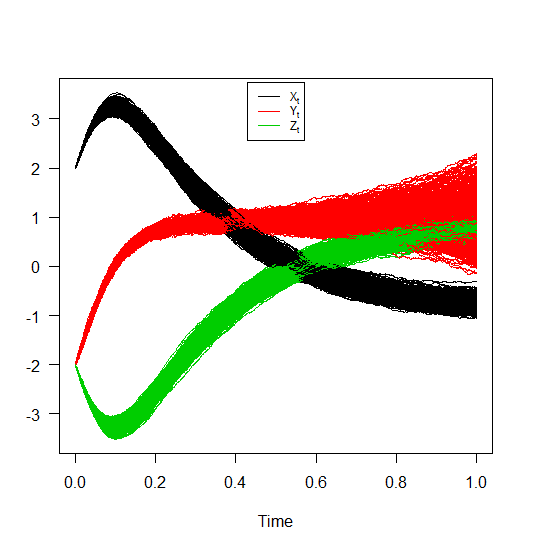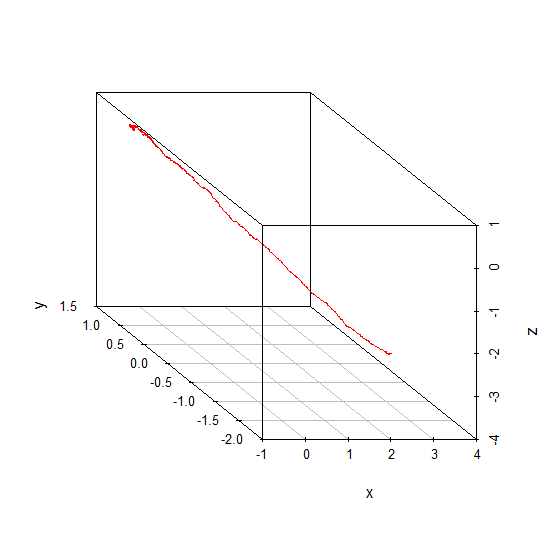Hence we can just make use of the rsde3d() function to build our random number for $$(X_{t},Y_{t},Z_{t})$$ at time $$t = 1$$.

R> out <- rsde3d(object = mod3d, at = 1)
R> head(out,n=3)
         x       y       z
1 -0.73929 0.65851 0.64650
2 -0.63795 0.41731 0.79531
3 -0.80249 1.16501 0.83721

For each SDE type and for each numerical scheme, the marginal density of $$X_t$$, $$Y_t$$ and $$Z_t$$ at time $$t=1$$ are reported using dsde3d() function, see e.g. Figure 8.

R> den <- dsde3d(mod3d,pdf="M",at =1)
R> plot(den, main="Marginal Density")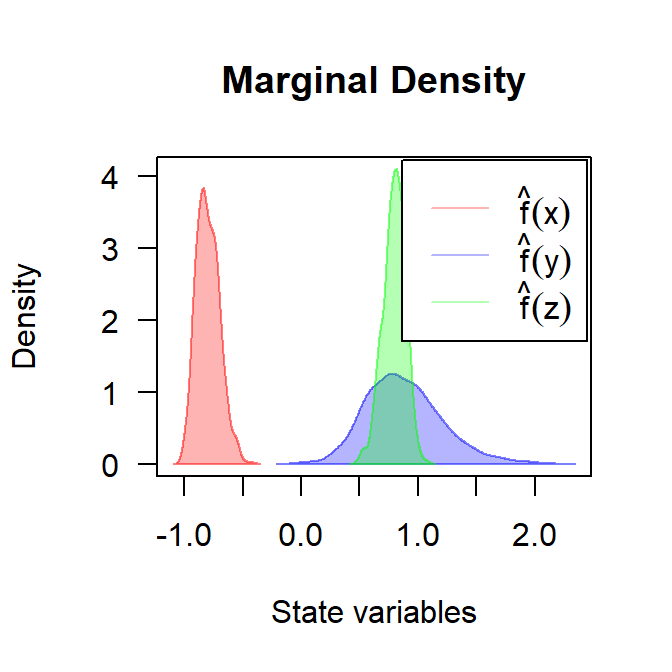Marginal density of $$X_t$$, $$Y_t$$ and $$Z_t$$ at time $$t=1$$

For an approximate joint transition density for $$(X_t,Y_t,Z_t)$$ (for more details, see package sm or ks.)

R> denJ <- dsde3d(mod3d,pdf="J")
R> plot(denJ,display="rgl")

## Attractive model for 3D diffusion processes

If we assume that $$U_w( x , y , z , t )$$, $$V_w( x , y , z , t )$$ and $$S_w( x , y , z , t )$$ are neglected and the dispersion coefficient $$D( x , y , z )$$ is constant. A system becomes (see Boukhetala,1996): $\begin{eqnarray}\label{eq19} % \nonumber to remove numbering (before each equation) \begin{cases} dX_t = \left(\frac{-K X_{t}}{\sqrt{X^{2}_{t} + Y^{2}_{t} + Z^{2}_{t}}}\right) dt + \sigma dW_{1,t} \nonumber\\ dY_t = \left(\frac{-K Y_{t}}{\sqrt{X^{2}_{t} + Y^{2}_{t} + Z^{2}_{t}}}\right) dt + \sigma dW_{2,t} \\ dZ_t = \left(\frac{-K Z_{t}}{\sqrt{X^{2}_{t} + Y^{2}_{t} + Z^{2}_{t}}}\right) dt + \sigma dW_{3,t} \nonumber \end{cases} \end{eqnarray}$ with initial conditions $$(X_{0},Y_{0},Z_{0})=(1,1,1)$$, by specifying the drift and diffusion coefficients of three processes $$X_{t}$$, $$Y_{t}$$ and $$Z_{t}$$ as R expressions which depends on the three state variables (x,y,z) and time variable t, with integration step size Dt=0.0001.

R> set.seed(1234, kind = "L'Ecuyer-CMRG")
R> K = 4; s = 1; sigma = 0.2
R> fx <- expression( (-K*x/sqrt(x^2+y^2+z^2)) , (-K*y/sqrt(x^2+y^2+z^2)) , (-K*z/sqrt(x^2+y^2+z^2)) )
R> gx <- rep(expression(sigma),3)
R> mod3d <- snssde3d(drift=fx,diffusion=gx,N=10000,x0=c(x=1,y=1,z=1))

The results of simulation (3D) are shown in Figure 9:

R> plot3D(mod3d,display="persp",col="blue")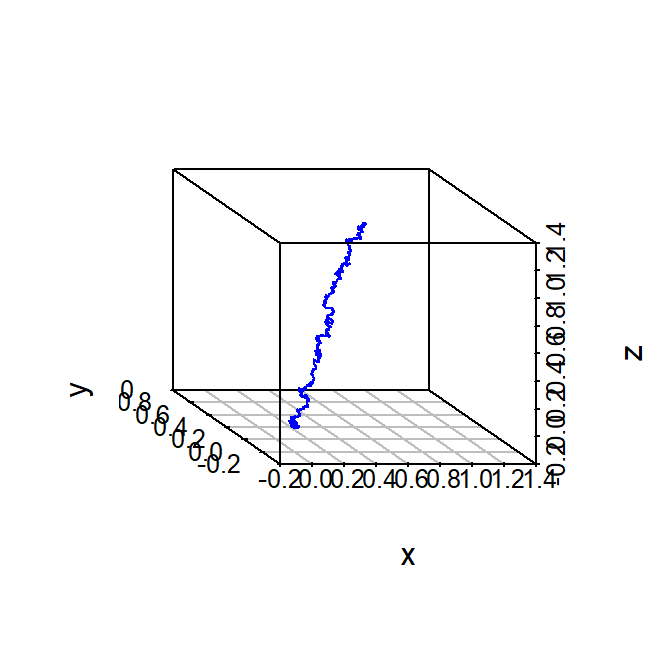Attractive model for 3D diffusion processes

## Transformation of an SDE one-dimensional

Next is an example of one-dimensional SDE driven by three correlated Wiener process ($$B_{1,t}$$,$$B_{2,t}$$,$$B_{3,t}$$), as follows: $\begin{equation}\label{eq20} dX_{t} = B_{1,t} dt + B_{2,t} dB_{3,t} \end{equation}$ with: $\Sigma= \begin{pmatrix} 1 & 0.2 &0.5\\ 0.2 & 1 & -0.7 \\ 0.5 &-0.7&1 \end{pmatrix}$ To simulate the solution of the process $$X_t$$, we make a transformation to a system of three equations as follows: $\begin{eqnarray}\label{eq21} \begin{cases} % \nonumber to remove numbering (before each equation) dX_t = Y_{t} dt + Z_{t} dB_{3,t} \nonumber\\ dY_t = dB_{1,t} \\ dZ_t = dB_{2,t} \nonumber \end{cases} \end{eqnarray}$ run by calling the function snssde3d() to produce a simulation of the solution, with $$\mu = 1$$ and $$\sigma = 1$$.

R> set.seed(1234, kind = "L'Ecuyer-CMRG")
R> fx <- expression(y,0,0)
R> gx <- expression(z,1,1)
R> Sigma <-matrix(c(1,0.2,0.5,0.2,1,-0.7,0.5,-0.7,1),nrow=3,ncol=3)
R> modtra <- snssde3d(drift=fx,diffusion=gx,M=1000,corr=Sigma)
R> modtra
Itô Sde 3D:
| dX(t) = Y(t) * dt + Z(t) * dB1(t)
| dY(t) = 0 * dt + 1 * dB2(t)
| dZ(t) = 0 * dt + 1 * dB3(t)
| Correlation structure:
1.0  0.2  0.5
0.2  1.0 -0.7
0.5 -0.7  1.0
Method:
| Euler scheme with order 0.5
Summary:
| Size of process   | N  = 1001.
| Number of simulation  | M  = 1000.
| Initial values    | (x0,y0,z0) = (0,0,0).
| Time of process   | t in [0,1].
| Discretization    | Dt = 0.001.

The histogram and kernel density of $$X_t$$ at time $$t=1$$ are reported using rsde3d() function, and we calculate emprical variance-covariance matrix $$C(s,t)=\text{Cov}(X_{s},X_{t})$$, see e.g. Figure 10.

R> X <- rsde3d(modtra,at=1)$x R> MASS::truehist(X,xlab = expression(X[t==1]));box() R> lines(density(X),col="red",lwd=2) R> legend("topleft",c("Distribution histogram","Kernel Density"),inset =.01,pch=c(15,NA),lty=c(NA,1),col=c("cyan","red"), lwd=2,cex=0.8) R> ## Cov-Matrix R> color.palette=colorRampPalette(c('white','green','blue','red')) R> filled.contour(time(modtra), time(modtra), cov(t(modtra$X)), color.palette=color.palette,plot.title = title(main = expression(paste("Covariance empirique:",cov(X[s],X[t]))),xlab = "time", ylab = "time"),key.title = title(main = ""))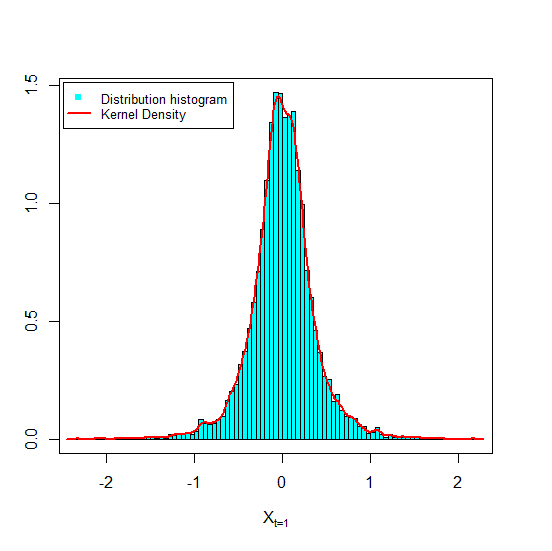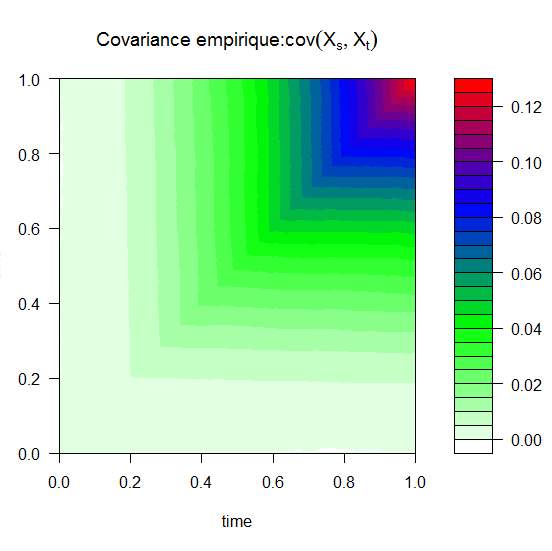3. The equivalently of $$X_{t}^{\text{mod1}}$$ the following Stratonovich SDE: $$dX_{t} = \theta X_{t} \circ dW_{t}$$.↩︎